﻿ 基于变步长蚁群算法的移动机器人路径规划
«上一篇文章快速检索 高级检索

 智能系统学报2021, Vol. 16Issue (2): 330-337  DOI: 10.11992/tis.2020040110

### 引用本文XU Yuqiong, LOU Ke, LI Zhikun. Mobile robot path planning based on variable-step ant colony algorithm[J]. CAAI Transactions on Intelligent Systems, 2021, 16(2): 330-337. DOI: 10.11992/tis.202004011.### 文章历史

1. 广州大学松田学院 电气与汽车工程系，广东 广州 511370;
2. 高端装备先进感知与智能控制教育部重点实验室，安徽 芜湖 241000;
3. 安徽工程大学 安徽省电气传动与控制重点实验室，安徽 芜湖 241000

Mobile robot path planning based on variable-step ant colony algorithm
XU Yuqiong 1,2,3, LOU Ke 2,3, LI Zhikun 2,3
1. Department of Electrical and Automotive Engineering, Songtian College, Guangzhou University, Guangzhou 511370, China;
2. Key Laboratory of Advanced Perception and Intelligent Control of High-End Equipment, Ministry of Education, Wuhu 241000, China;
3. Anhui Provincial Key Laboratory of Electric Transmission and Control, Anhui Polytechnic University, Wuhu 241000, China
Abstract: To address the problems of the traditional and double-layer ant colony algorithms, such as their low search efficiency, slow convergence, and high path–planning cost, in this paper we propose a variable-step ant colony algorithm. The proposed algorithm expands the set of mobile locations of the ant colony, and uses the variable-step strategy of selecting the hopping points, thus effectively shortening the path length of the mobile robot. The initialization pheromone adopts an uneven distribution, which increases the pheromone concentration of the grid in a straight line from the start to end points, with the pheromone decaying outward in parallel. The heuristic information matrix is improved and the method used to calculate the heuristic function of the mobile robot from the current to the end positions is adjusted. The experimental results show that the performance of the variable-step ant colony algorithm is superior to those of the double-layer and traditional ant colony algorithms with respect to path length and convergence speed, which proves its effectiveness and superiority. Thus, the proposed algorithm is effective in solving the path-planning problem of mobile robots.
Key words: traditional ant colony algorithm    double-layer ant colony algorithm    path planning    variable-step    pheromone    heuristic function    convergence    mobile robot

1 蚁群算法原理 1.1 环境表示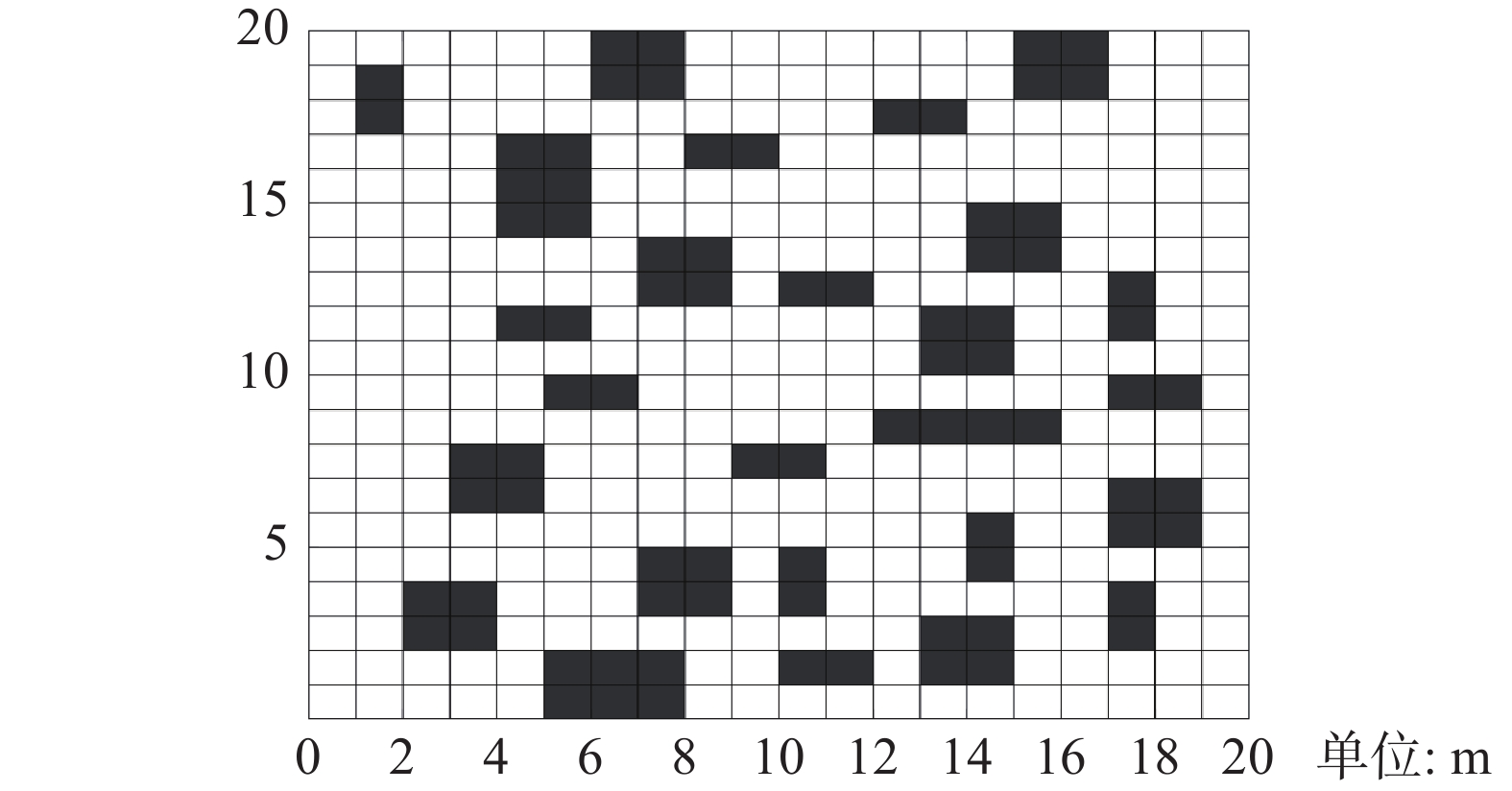Download: 图 1 栅格地图 Fig. 1 Raster map
1.2 搜索方式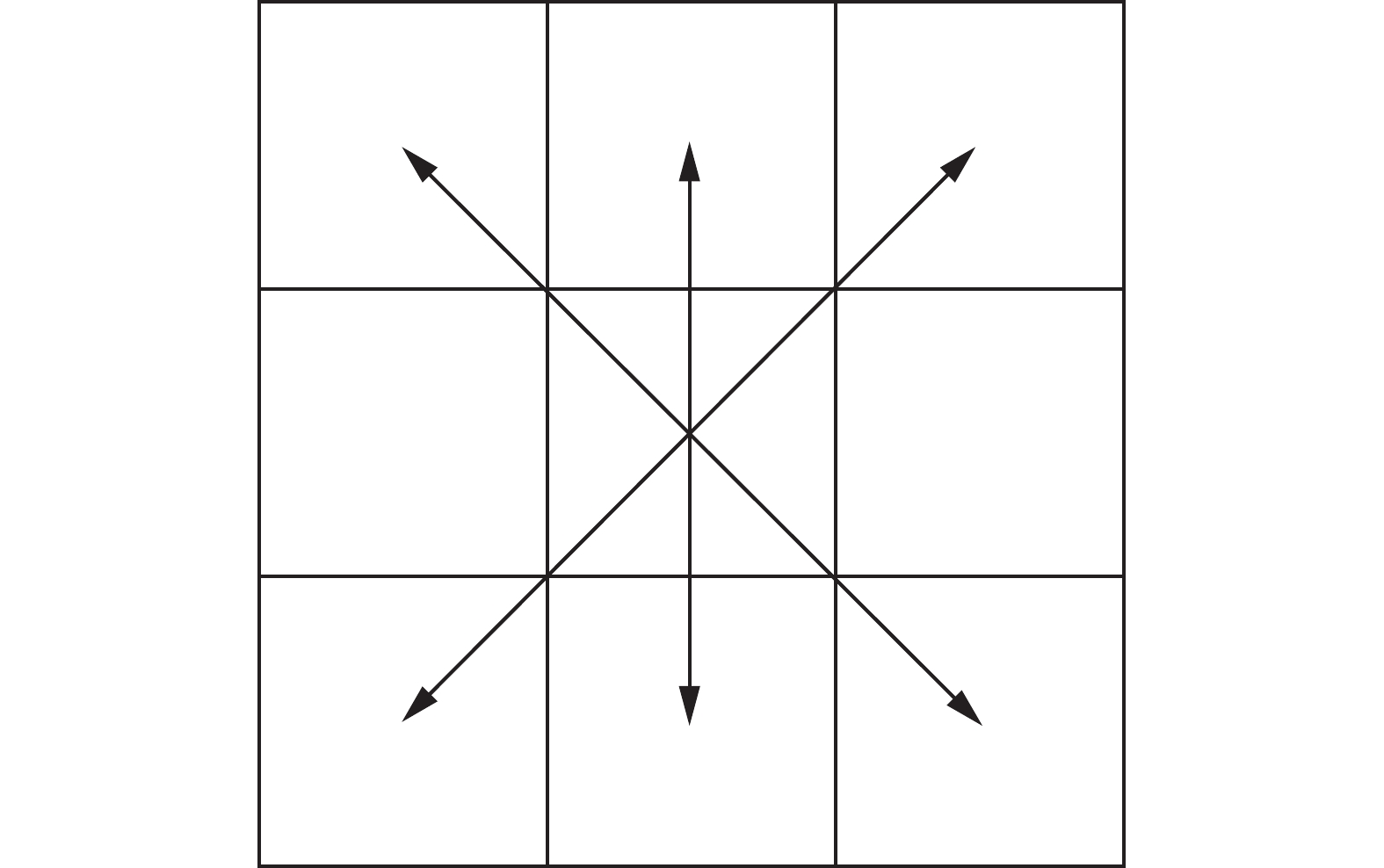Download: 图 2 移动方式 Fig. 2 Moving method

 $p_{ij}^k(t) = \left\{ {\begin{array}{*{20}{l}} {\dfrac{{{{[{\tau _{ij}}(t)]}^\alpha } \cdot {{[{\eta _{ij}}(t)]}^\beta }}}{{\displaystyle\sum \limits_{s \in {a_{_k}}} {{[{\tau _{is}}(t)]}^\alpha } \cdot {{[{\eta _{{\rm{is}}}}(t)]}^\beta }}},}\quad{j \in {a_k}}\\ {0,}\quad{\text{其他}} \end{array}} \right.$ (1)
 ${\eta _{ij}} = \frac{1}{{{d_{ij}}}}$ (2)

 ${\tau _{ij}} = (1 - \rho ){\tau _{ij}}$ (3)

 ${\tau _{ij}} = {\tau _{ij}} + \sum\limits_{k = 1}^m {\Delta \tau _{ij}^k}$ (4)

 $\Delta \tau _{ij}^k = \left\{ {\begin{array}{*{20}{l}} {1/{d_{ij}},}\quad{{\text{边}}(i,j){\text{在路径}}{T^{{k}}}{\text{上}}}\\ {{\rm{0,}}}\quad{\text{其他}} \end{array}} \right.$ (5)

2 变步长蚁群算法

2.1 步长选择策略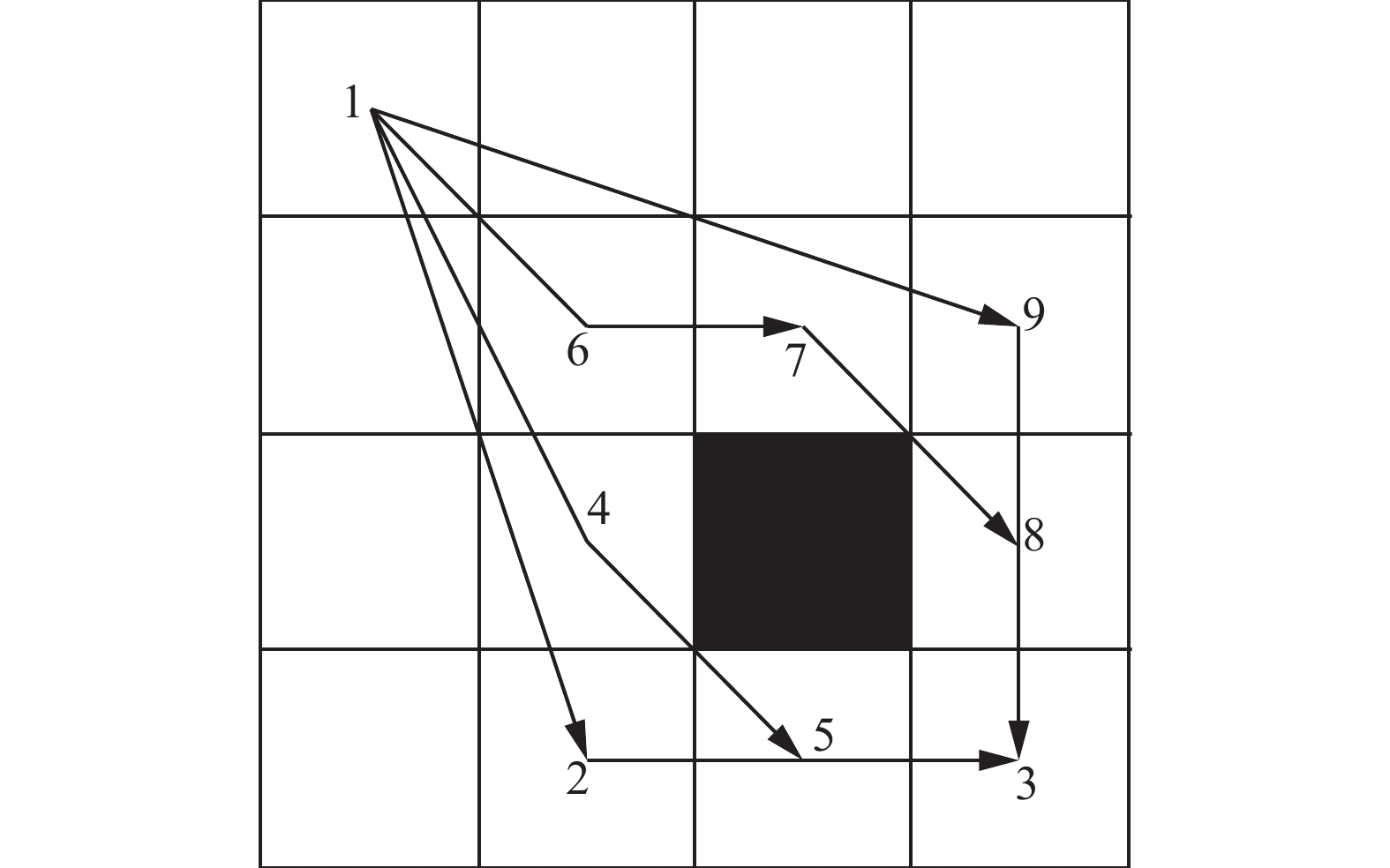Download: 图 3 步长选择 Fig. 3 Step size selection

 $p_{ij}^k(t) = \left\{ {\begin{array}{*{20}{l}} {k \cdot \phi \cdot \mu \cdot \dfrac{{{{[{\tau _{ij}}(t)]}^\alpha } \cdot {{[{\eta _{ij}}(t)]}^\beta }}}{{\displaystyle\sum\limits_{s \in {a_k}} {{{[{\tau _{is}}(t)]}^\alpha }{{[{\eta _{is}}(t)]}^\beta }} }},}\quad{j \in {a_k}}\\ {{\rm{0,}}}\quad{\text{其他}} \end{array}} \right.$ (6)
 $\phi {\rm{ = }}\dfrac{{\displaystyle\sum\limits_{{{s}} \in {a_k}} {{{[{\gamma _{is}}(t)]}^\varepsilon }} }}{{{\sigma ^\varepsilon }}}$ (7)
 $\mu = \dfrac{{\displaystyle\sum\limits_{s \in {a_k}} {{{[{\gamma _{is}}(t)]}^\omega }} }}{{{\lambda ^\omega }}}$ (8)

2.2 信息素分布策略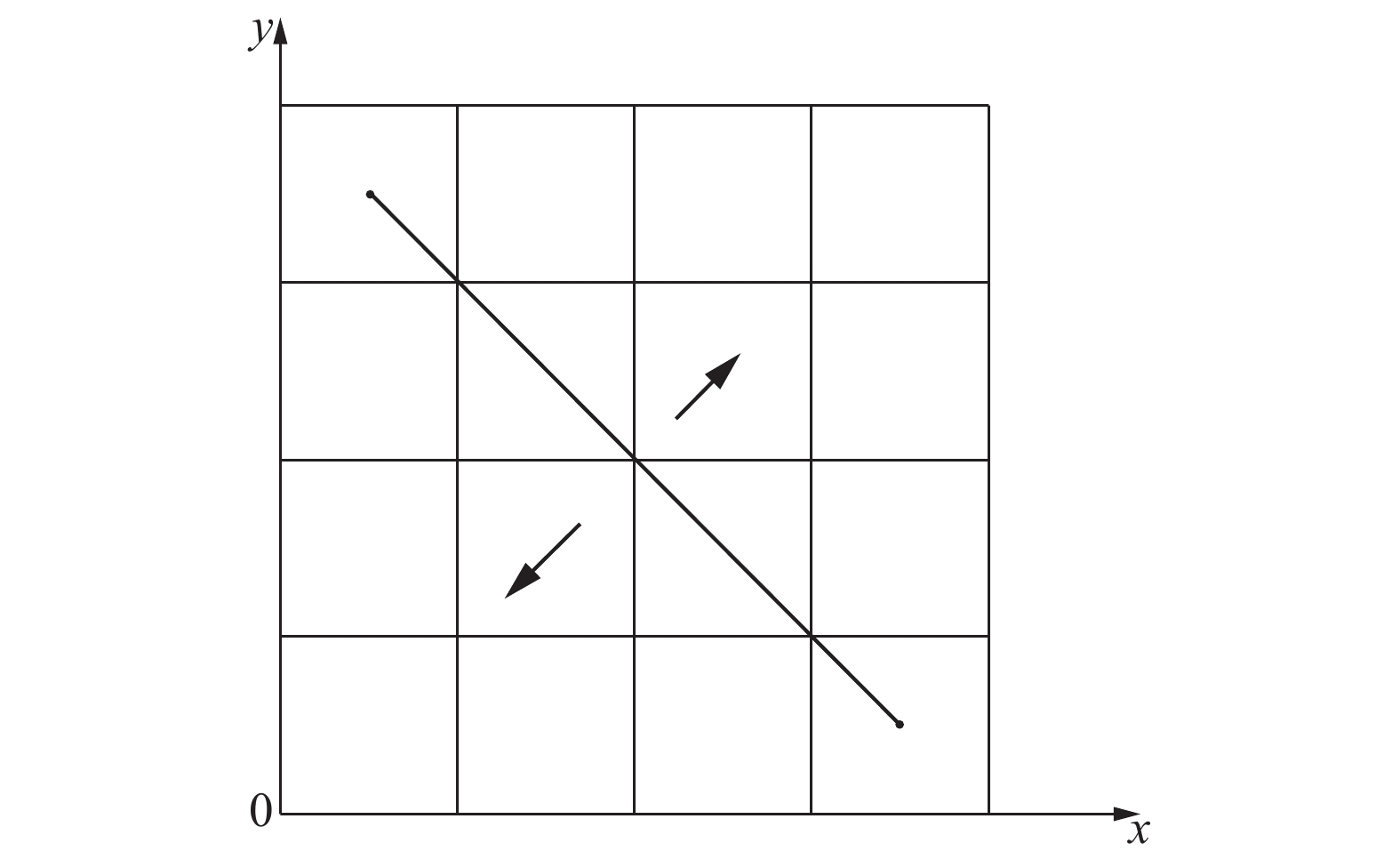Download: 图 4 信息素分布策略 Fig. 4 Pheromone distribution strategy

 $y = - x + C,\quad C \in {N^ * }$ (9)

 ${\tau _0} = \left\{ {\begin{array}{*{20}{l}} {T,}\quad{y = - x + C}\\ {\dfrac{{C - 1}}{C} \cdot T,}\quad{y = - x + C \pm 1}\\ {\dfrac{{C - 2}}{C} \cdot T,}\quad{y = - x + C \pm 2}\\ \cdots &{}\\ {\dfrac{1}{C} \cdot T,}\quad{y = - x + 1{\text{或}}y = - x + 2C - 1} \end{array}} \right.$ (10)

 ${\tau _{ij}}(t + 1) = (1 - \rho ) \cdot {\tau _{ij}}(t) + \Delta \tau _{_{ij}}^{{\rm{best}}}(t),\quad\rho \in (0,1)$ (11)
 $\Delta \tau _{ij}^{{\rm{best}}} = \left\{ {\begin{array}{*{20}{l}} {\dfrac{1}{{{L^{{\rm{best}}}}}},}\quad{{\text{边}}(i,j){\text{包含在最优路径内}}}\\ {{\rm{0}},}\quad{\text{其他}} \end{array}} \right.$ (12)

2.3 改进启发函数

 ${\eta _{{{ij}}}} = \frac{1}{{{d_{ij}}}} \cdot \frac{{\sqrt 2 }}{{|{x_i} + {y_j} - C|}}$ (13)

3 改进算法步骤

1) 针对各项相关参数进行初始化：路径规划的起始点及终点、信息素重要程度参数 $\alpha$ 、启发因子重要程度参数 $\beta$ 、迭代次数、蚂蚁数量、信息素挥发系数及增强系数等相关参数，建立地图二维栅格模型，邻接矩阵模型。

2) 初始化信息素采用不均匀分布，加强起点至终点直线所涉及栅格的信息素浓度，平行的向外衰减；改进启发式信息矩阵，调整移动机器人当前位置到终点位置的启发函数计算方法，建立启发式信息矩阵。

3) 建立禁忌表以及对禁忌表初始化，将起点、障碍物节点、引起死锁的节点均加入禁忌表中。

4) 计算启发信息，根据信息素浓度以及概率公式确定蚂蚁下一步可以到达的节点，记录路径并更新，更新禁忌表。

5) 当所有蚂蚁完成一次迭代后，保存最优路径，更新信息素及禁忌表，如果没有完成一次迭代，则继续开始下一只蚂蚁路径寻优。

6) 如果蚂蚁完成所有迭代次数，则输出最优路径及收敛迭代次数，如果没有完成所有迭代次数，则继续开始下一次迭代。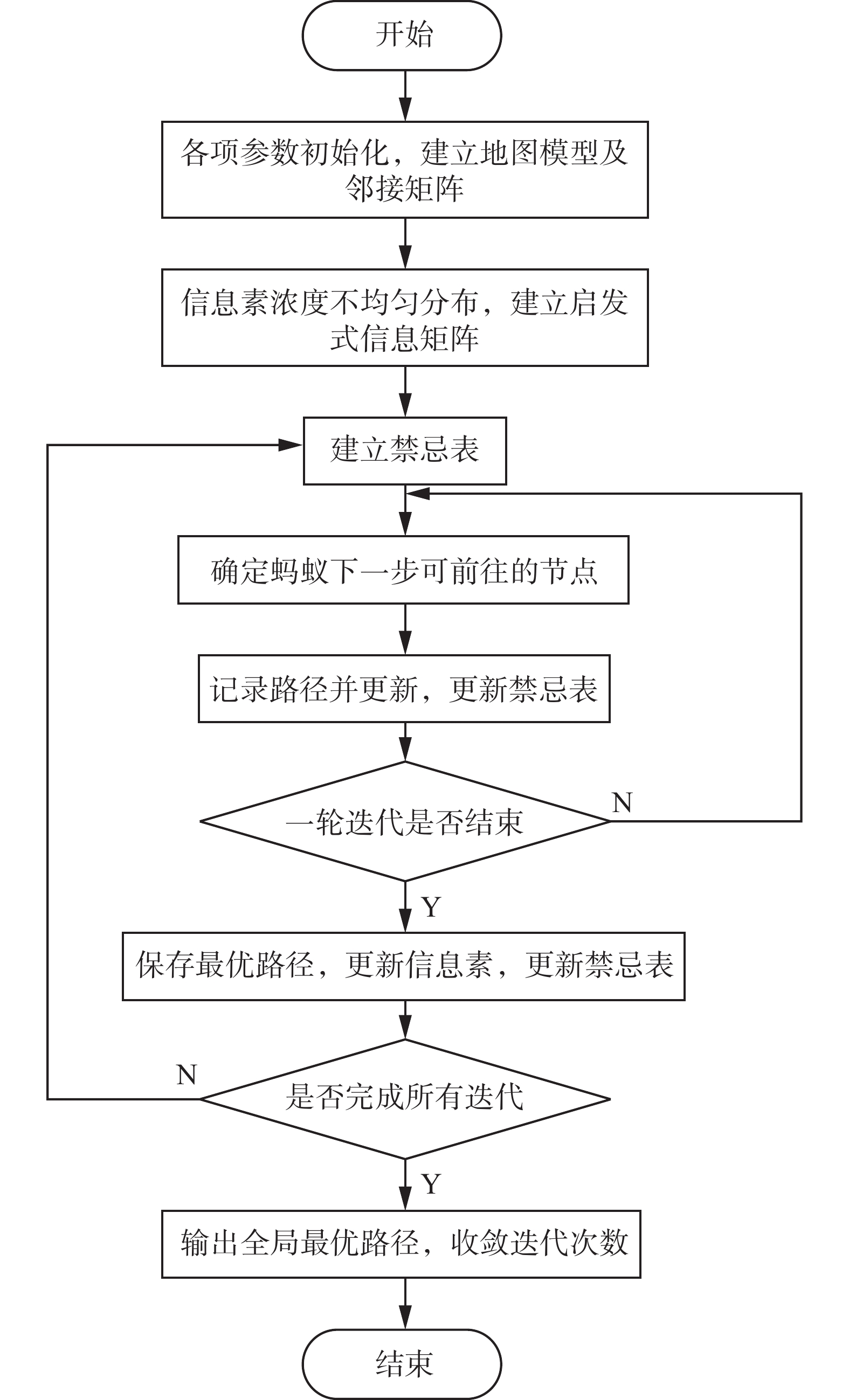Download: 图 5 变步长蚁群算法流程 Fig. 5 Flow chart of variable step size ant colony algorithm
4 算法仿真

4.1 $20 \times 20$ 栅格环境

$20 \times 20$ 的栅格环境下，对传统蚁群算法和本文算法进行仿真，其中，路径规划仿真分别如图67所示，实验数据如表1所示，传统蚁群算法路径规划长度为30.870 m，文献算法路径规划长度为32.1421 m，本文算法路径规划长度为28.042 m，显然，本文算法在路径规划方面优于文献算法及传统蚁群算法，本文算法较文献算法、传统蚁群算法的路径长度分别缩短了12.76%及9.16%。本文算法提出信息素不均匀分布策略，提高了最优路径上节点被选择的概率，通过节点距离起点至终点对角线的距离，改进启发函数，提高了算法的全局搜索能力，有效缩短了全局最优路径的长度。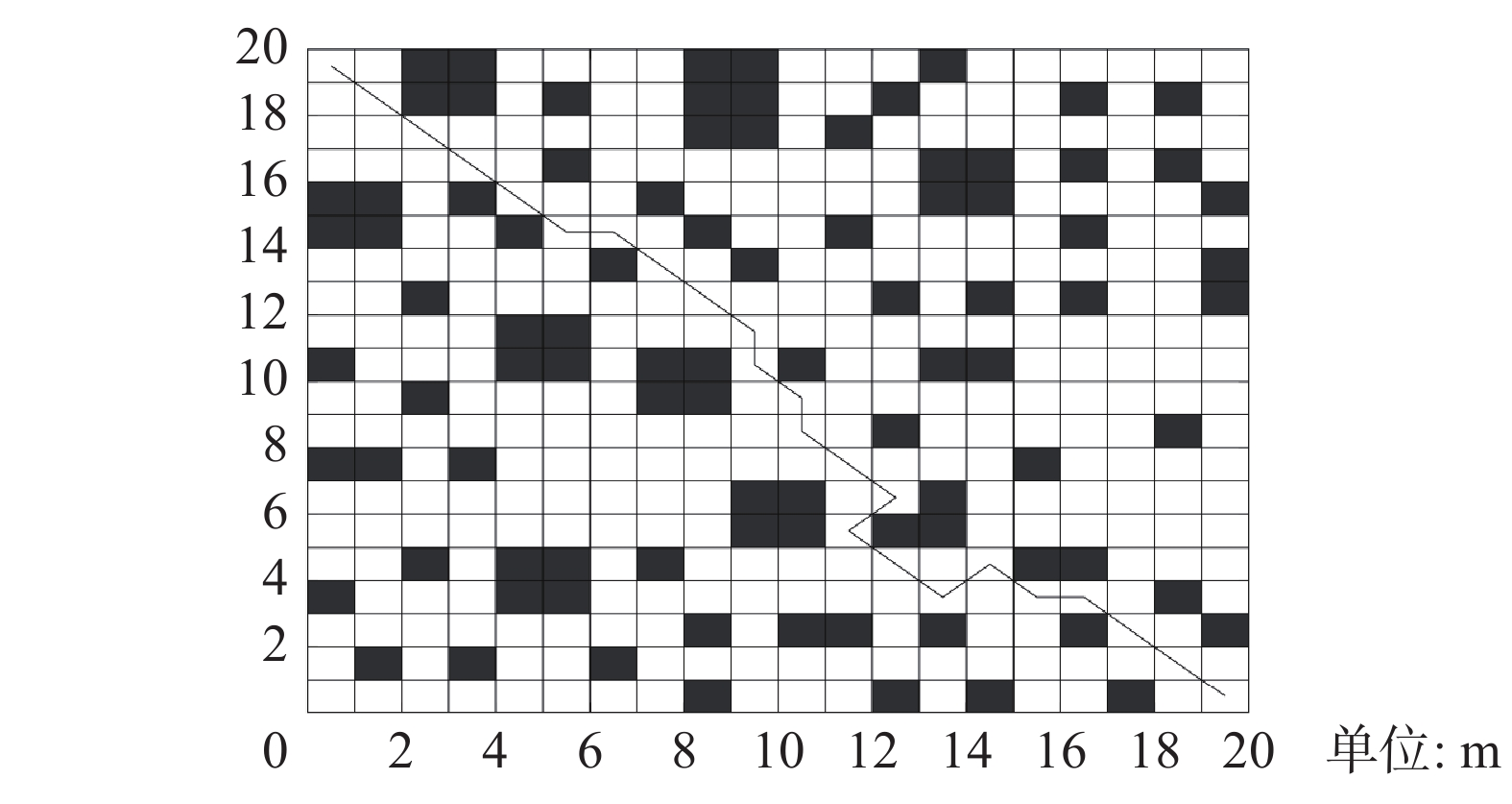Download: 图 6 传统蚁群算法路径规划(20×20) Fig. 6 Path planning of traditional ant colony algorithm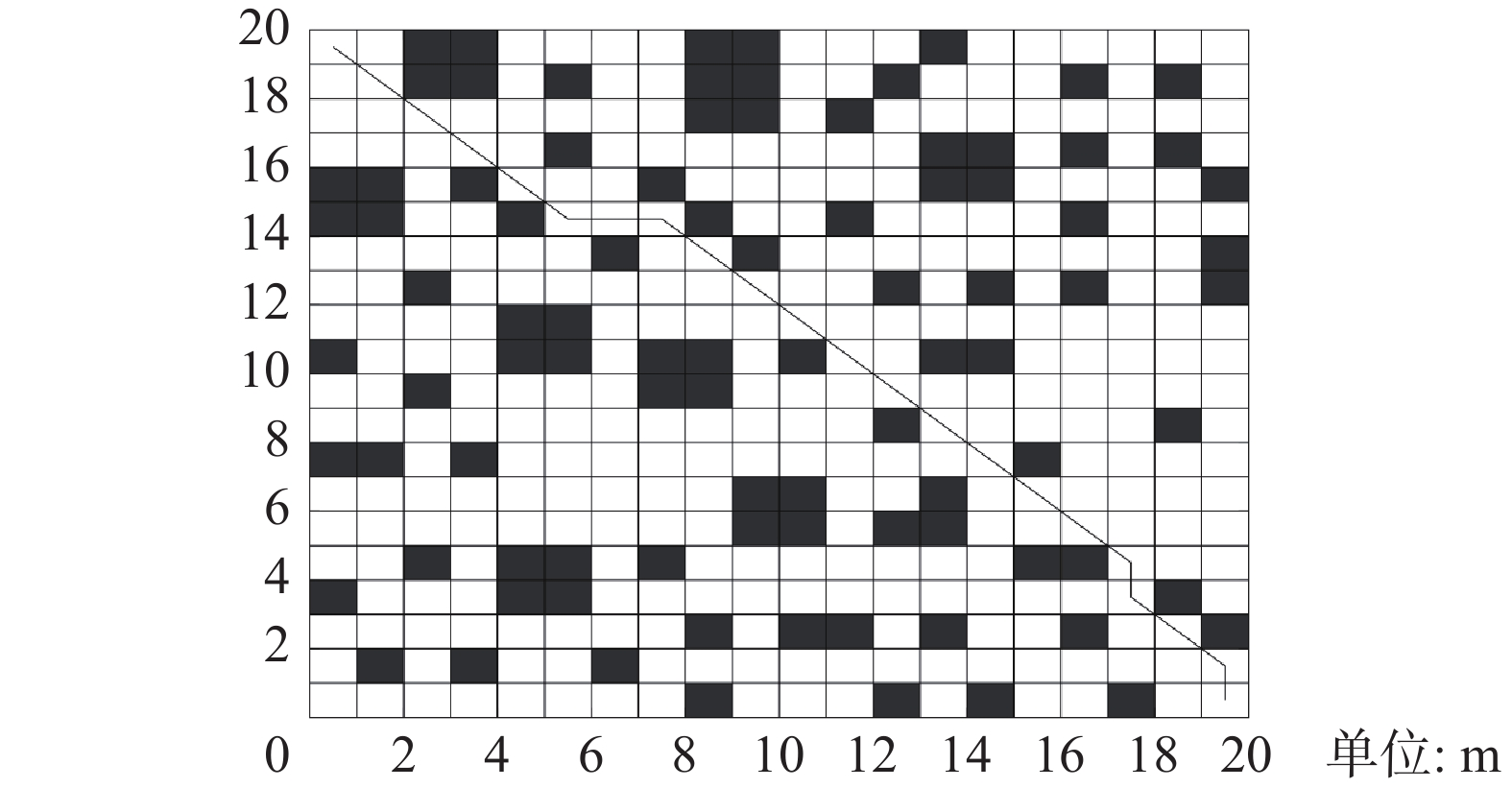Download: 图 7 本文算法路径规划(20×20) Fig. 7 Algorithm path planning in this paper表 1 各算法实验结果对比 Tab.1 Comparison of experimental results of various algorithms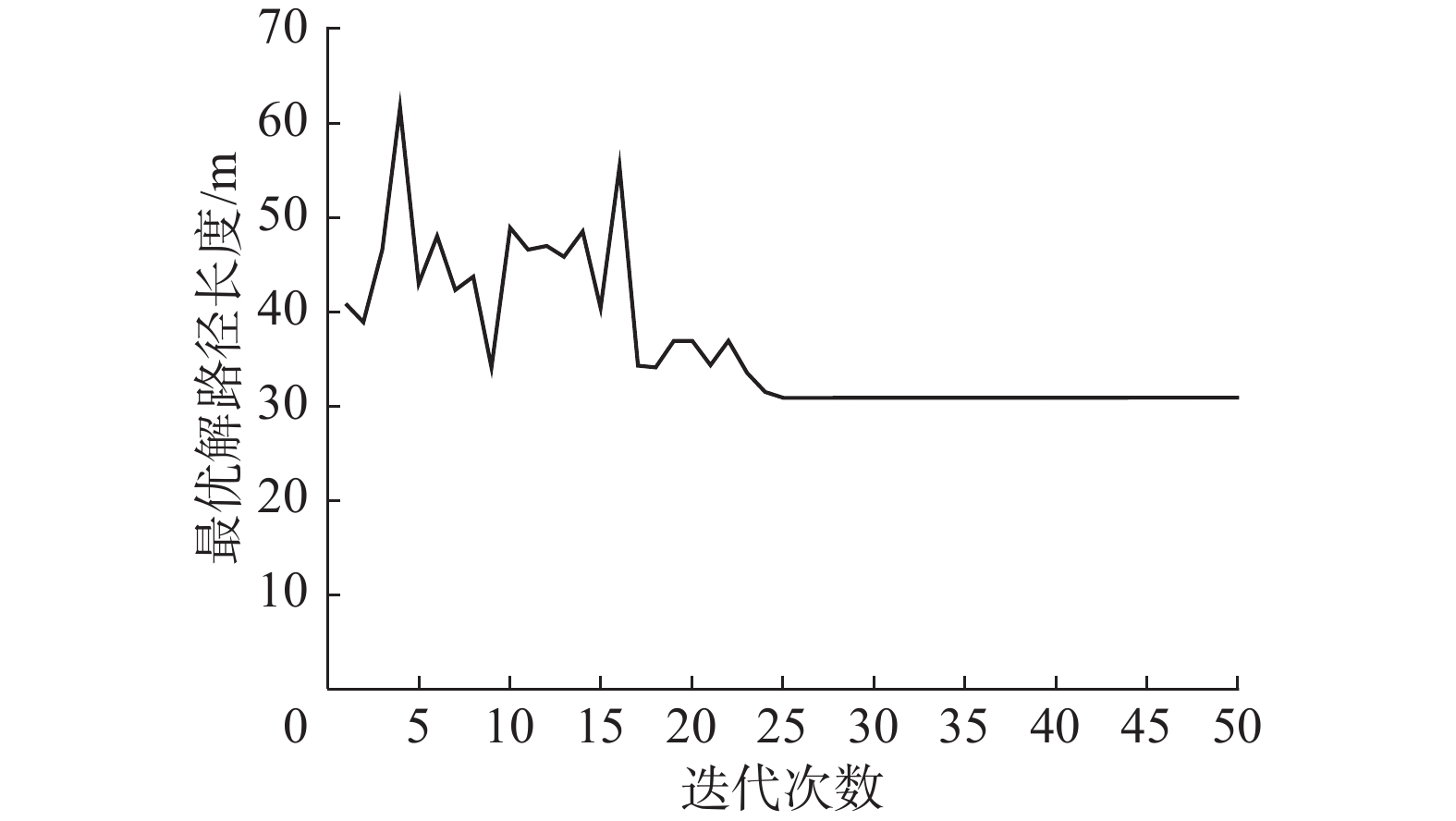Download: 图 8 传统蚁群算法收敛曲线 Fig. 8 Convergence curve of traditional ant colony algorithm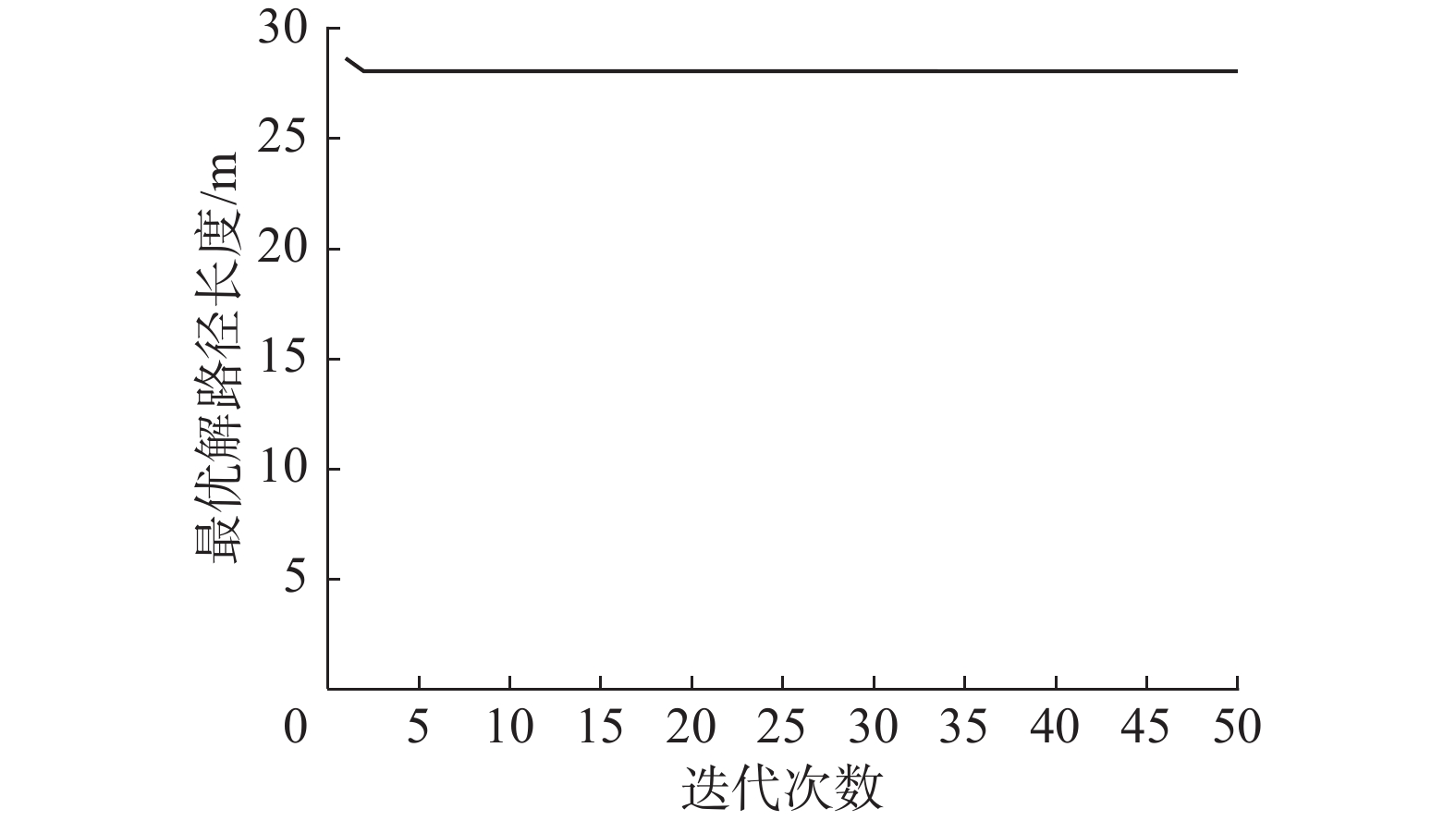Download: 图 9 本文算法收敛曲线 Fig. 9 Convergence curve of the algorithm presented in this paper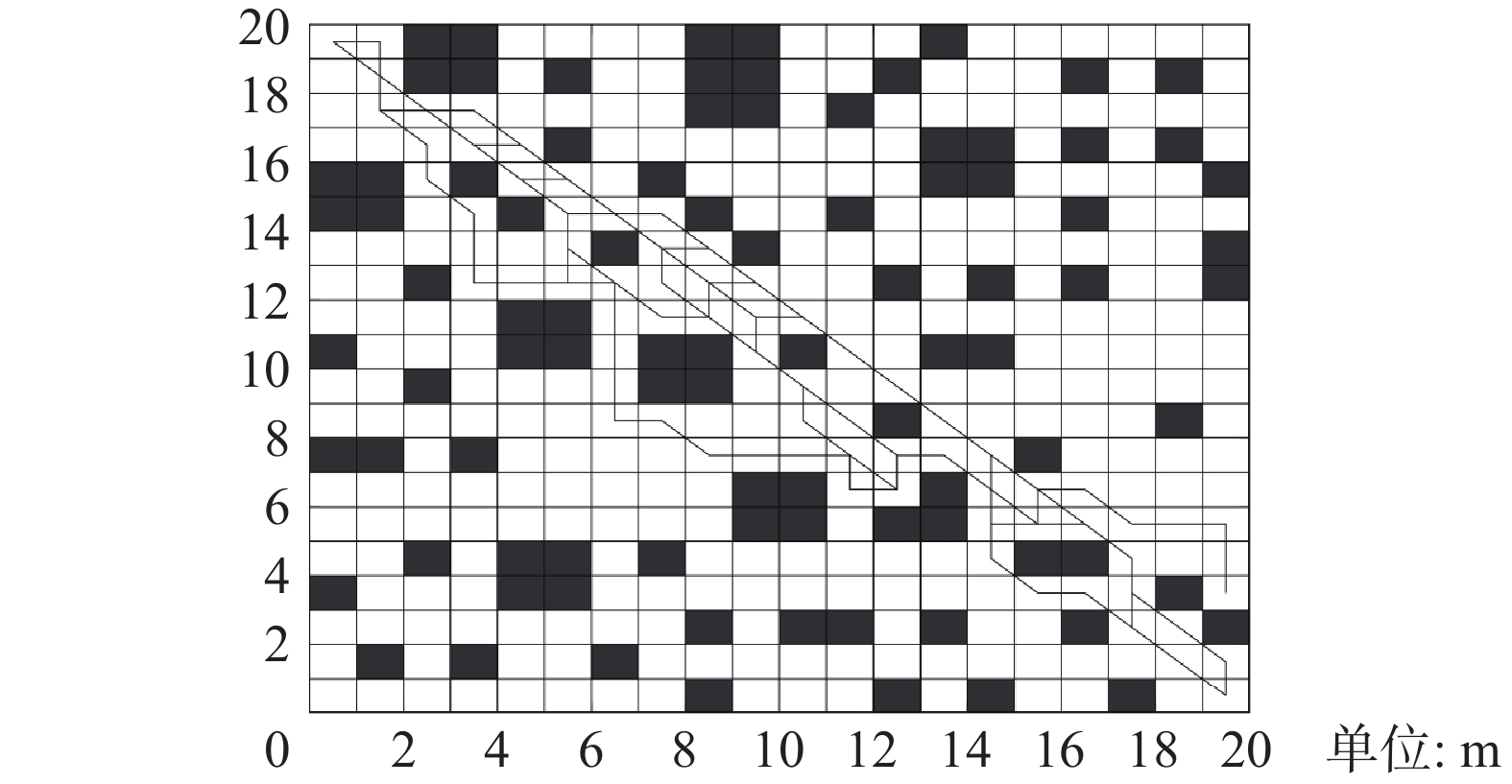Download: 图 10 本文算法各代蚁群最优路径规划(20×20) Fig. 10 Optimal path planning of each generation of ant colony in this algorithm (20×20)
4.2 $30 \times 30$ 栅格环境

$30 \times 30$ 栅格环境下，采用文献中的同一个栅格地图，对本文算法进行仿真实验，实验数据如表2所示，本文算法的路径规划如图11所示，本文算法的最佳路径长度为41.961 m，文献的最佳路径长度为45.1127 m，本文算法较文献关于最佳路径长度缩短了6.99%，因此，在复杂栅格环境下，本文算法依然保持良好的路径规划能力，通过变步长蚁群算法，有效地缩短了最优路径长度，收敛迭代效率如图12所示，本文算法的收敛迭代次数为6次，文献算法的收敛迭代次数为8次，本文算法较文献算法关于收敛迭代次数减少了25%，栅格地图越复杂，本文算法的优越性越明显，各代最优路径规划路线如图13所示，各代路径规划的路线依然集中于起点至终点的连线处，移动机器人将快速的寻找出最优路径。表 2 两种算法实验结果对比 Tab.2 Comparison of experimental results of various algorithms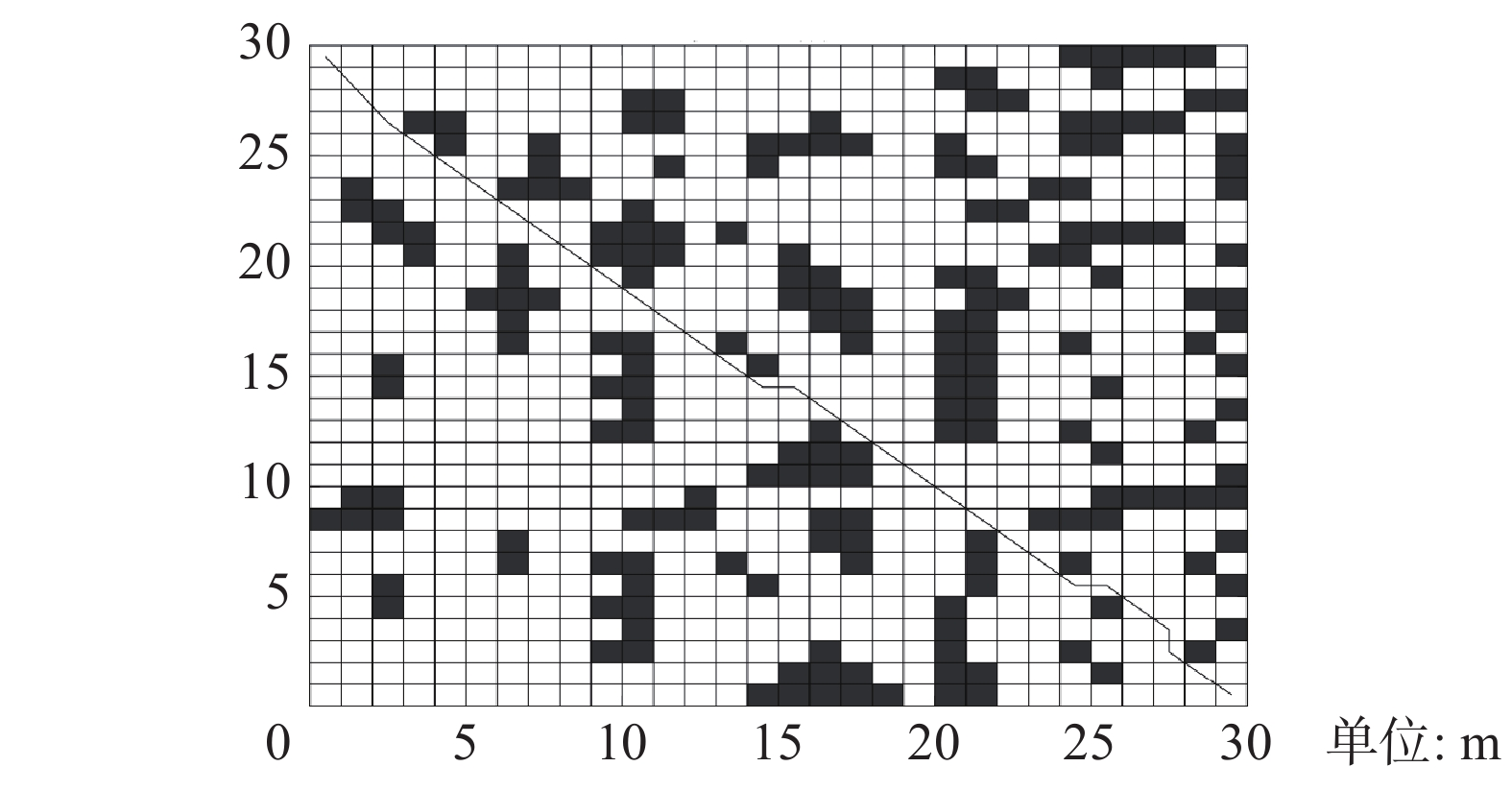Download: 图 11 本文算法路径规划(30×30) Fig. 11 Algorithm path planning in this paper (30×30)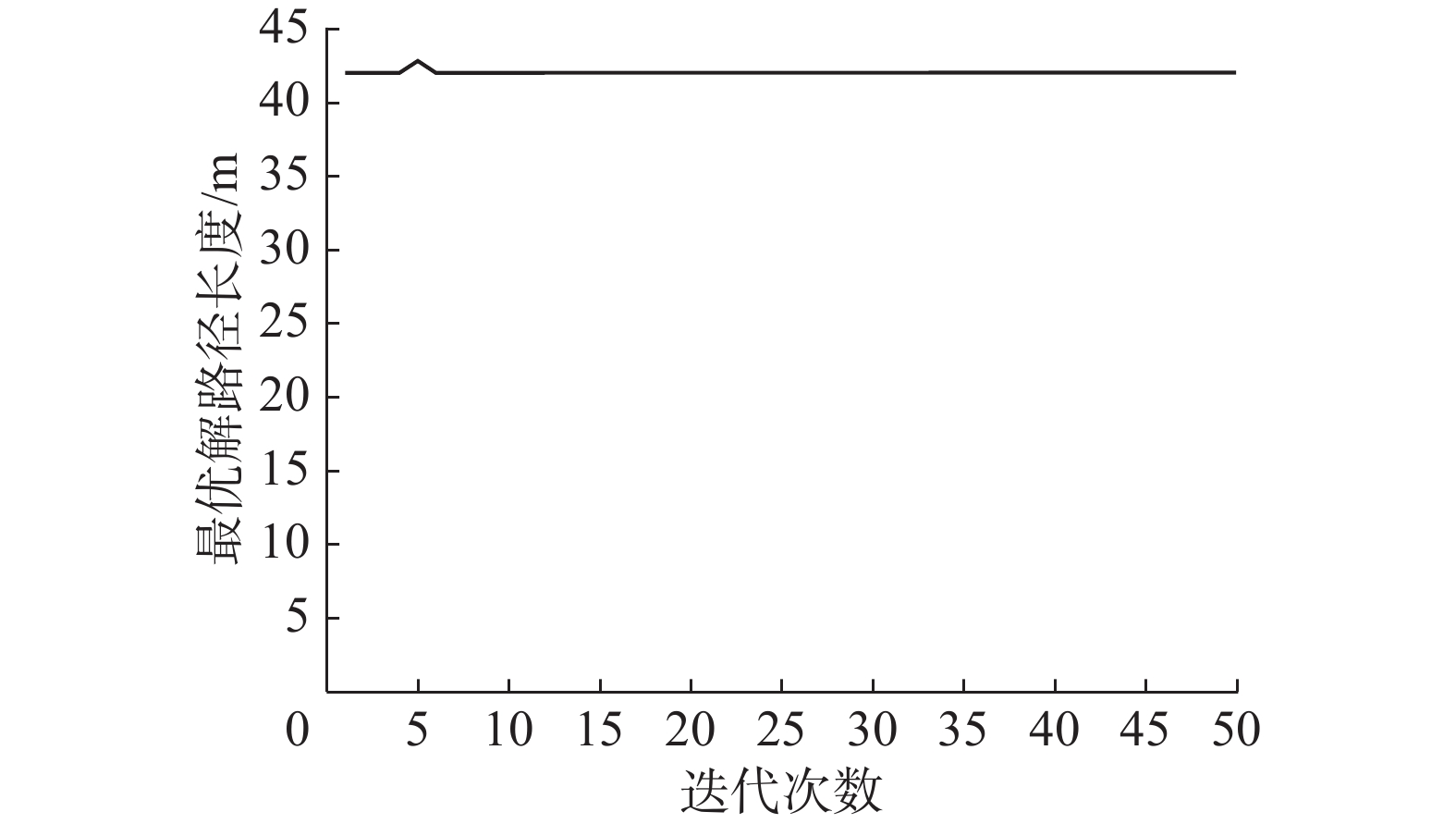Download: 图 12 本文算法收敛曲线(30×30) Fig. 12 Convergence curve of the algorithm presented in this paper (30×30)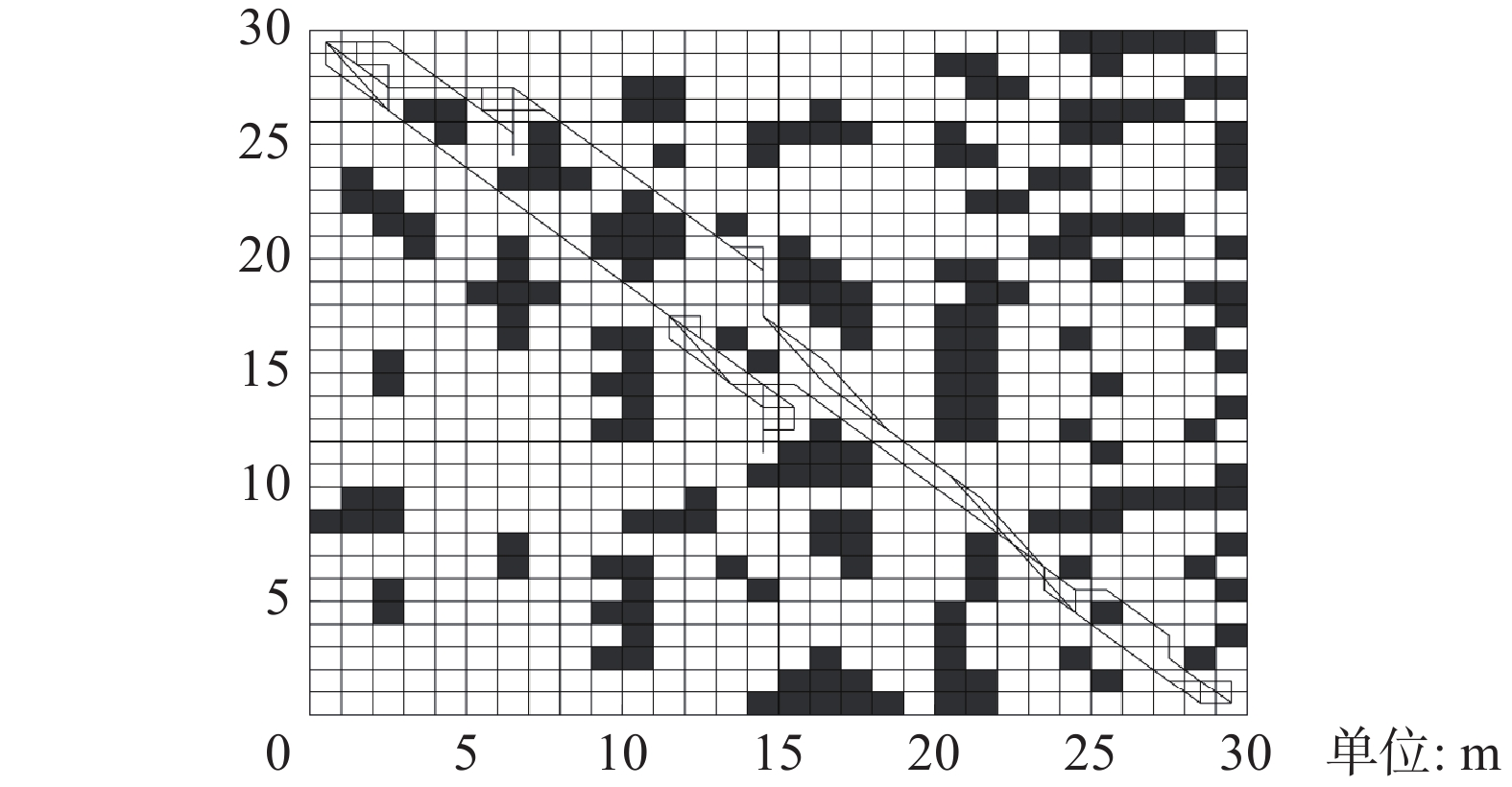Download: 图 13 本文算法各代蚁群最优路径规划(30×30) Fig. 13 Optimal path planning of each generation of ant colony in this algorithm (30×30)
4.3 实验研究Download: 图 14 移动机器人起点位置 Fig. 14 Starting position of mobile robotDownload: 图 15 移动机器人运行过程 Fig. 15 Mobile robot running process表 3 各算法路径规划实验数据 Tab.3 Experimental data of path planning for each algorithm
5 结束语

  CONFESSORE G, FABIANO M, LIOTTA G. A network flow based heuristic approach for optimising AGV movements[J]. Journal of intelligent manufacturing, 2013, 24(2): 405-419. DOI:10.1007/s10845-011-0612-7 (0)  张毅, 权浩, 文家富. 基于独狼蚁群混合算法的移动机器人路径规划[J]. 华中科技大学学报(自然科学版), 2020, 48(1): 127-132. ZHANG Yi, QUAN Hai, WEN Jiafu. Mobile robot path planning based on the wolf ant colony hybrid algorithm[J]. Journal of Huazhong University of Science and Technology (Nature Science Edition), 2020, 48(1): 127-132. (0)  刘可, 李可, 宿磊, 等. 基于蚁群算法与参数迁移的机器人三维路径规划方法[J]. 农业机械学报, 2020, 51(1): 29-36. LIU Ke, LI Ke, SU Lei, et al. Robot 3D path planning method based on ant colony algorithm and parameter transfer[J]. Transactions of the Chinese society for agricultural machinery, 2020, 51(1): 29-36. (0)  ZHOU Zhiping, NIE Yunfeng, GAO Min. Enhanced ant colony optimization algorithm for global path planning of mobile robots[C]//Proceedings of 2013 International Conference on Computational and Information Sciences. Shiyang, China, 2013: 698−701. (0)  HE Qiang, HU Xiangtao, REN Hong, et al. A novel artificial fish swarm algorithm for solving large-scale reliability-redundancy application problem[J]. ISA transactions, 2015, 59: 105-113. DOI:10.1016/j.isatra.2015.09.015 (0)  WU Tao, JING Xiaojun. Exploration of multiple access interference suppression based on multi-user detection[J]. Chinese journal of electronics, 2019, 28(4): 835-840. DOI:10.1049/cje.2019.05.011 (0)  陈军章. 改进人工鱼群算法的机器人路径规划及跟踪[J]. 机械设计与制造, 2019(04): 251-255. CHEN Junzhang. Mobile Robot Path Planning and Tracking Based on Improved Artificial Fish Swarm Algorithm[J]. Machinery Design & Manufacture, 2019(04): 251-255. DOI:10.3969/j.issn.1001-3997.2019.04.062 (0)  许凯波, 鲁海燕, 黄洋, 等. 基于双层蚁群算法和动态环境的机器人路径规划方法[J]. 电子学报, 2019, 47(10): 2166-2176. XU Kaibo, LU Haiyan, HUANG Yang, et al. Robot path planning based on double-layer ant colony optimization algorithm and dynamic environment[J]. Acta electronica sinica, 2019, 47(10): 2166-2176. DOI:10.3969/j.issn.0372-2112.2019.10.019 (0)  朱艳, 游晓明, 刘升. 基于启发式机制的改进蚁群算法[J]. 信息与控制, 2019, 48(3): 265-271. ZHU Yan, YOU Xiaoming, LIU Sheng. Improved ant colony algorithm based on heuristic mechanism[J]. Information and control, 2019, 48(3): 265-271. (0)  LIU Jianhua, YANG Jianguo, LIU Huaping, et al. An improved ant colony algorithm for robot path planning[J]. Soft computing, 2017, 21(19): 5829-5839. DOI:10.1007/s00500-016-2161-7 (0)  HWU T, WANG A Y, OROS N, et al. Adaptive robot path planning using a spiking neuron algorithm with axonal delays[J]. IEEE transactions on cognitive and developmental systems, 2018, 10(2): 126-137. DOI:10.1109/TCDS.2017.2655539 (0)  封声飞, 雷琦, 吴文烈, 等. 自适应蚁群算法的移动机器人路径规划[J]. 计算机工程与应用, 2019, 55(17): 35-43. FENG Shengfei, LEI Qi, WU Wenlie, et al. Mobile robot path planning based on adaptive ant colony algorithm[J]. Computer Engineering and Applications, 2019, 55(17): 35-43. DOI:10.3778/j.issn.1002-8331.1903-0401 (0)  黄琰, 李岩, 俞建成, 等. AUV智能化现状与发展趋势[J]. 机器人, 2020, 42(2): 215-231. HUANG Yan, LI Yan, YU Jiancheng, et al. State-of-the-art and development trends of AUV intelligence[J]. Robot, 2020, 42(2): 215-231. (0)  江明, 王飞, 葛愿, 等. 基于改进蚁群算法的移动机器人路径规划研究[J]. 仪器仪表学报, 2019, 40(2): 113-121. JIANG Ming, WANG Fei, GE Yuan, et al. Research on path planning of mobile robot based on improved ant colony algorithm[J]. Chinese journal of scientific instrument, 2019, 40(2): 113-121. (0)  CAO Jingang. Robot global path planning based on an improved ant colony algorithm[J]. Journal of computer and communications, 2016, 4(2): 11-19. DOI:10.4236/jcc.2016.42002 (0)  徐玉琼, 娄柯, 李婷婷, 等. 改进自适应蚁群算法的移动机器人路径规划[J]. 电子测量与仪器学报, 2019, 33(10): 89-95. XU Yuqiong, LOU Ke, LI Tingting, et al. Path planning of mobile robot based on improved adaptive ant colony algorithm[J]. Journal of electronic measurement and instrumentation, 2019, 33(10): 89-95. (0)  AJEIL F H, IBRAHEEM I K, AZAR A T, et al. Grid-based mobile robot path planning using aging-based ant colony optimization algorithm in static and dynamic environments[J]. Sensors, 2020, 20(7): 1880. DOI:10.3390/s20071880 (0)  杨俊成, 李淑霞, 蔡增玉. 路径规划算法的研究与发展[J]. 控制工程, 2017, 24(7): 1473-1480. YANG Juncheng, LI Shuxia, CAI Zengyu. Research and development of path planning algorithm[J]. Control engineering of China, 2017, 24(7): 1473-1480. (0)  王培良, 张婷, 肖英杰. 蚁群元胞优化算法在人群疏散路径规划中的应用[J]. 物理学报, 2020, 69(8): 234-242. WANG Peiliang, ZHANG Ting, XIAO Yingjie. Application research of ant colony cellular optimization algorithm in population evacuation path planning[J]. Acta physica sinica, 2020, 69(8): 234-242. (0)  万方, 周风余, 尹磊, 等. 基于电势场法的移动机器人全局路径规划算法[J]. 机器人, 2019, 41(6): 742-750. WAN Fang, ZHOU Fengyu, YIN Lei, et al. Global path planning algorithm of mobile robot based on electric potential field[J]. Robot, 2019, 41(6): 742-750. (0)